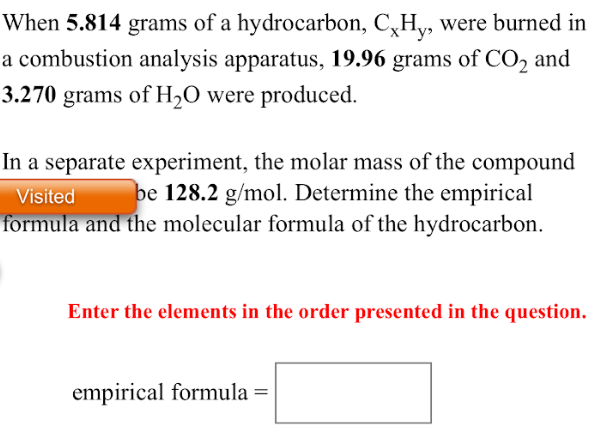# When 5.814 grams of a hydrocarbon, CxHy, were burned in a combustion analysis apparatus, 19.96 grams of CO2 and 3.270 grams of H2O were produced. In a separate experiment, the molar mass of the compound was found to be 128.2g/mol. Determine the empirical formula and the molecular formula and the molecular formula of the hydrocarbon. Enter the elements in the order presented in the question. Empirical formula=